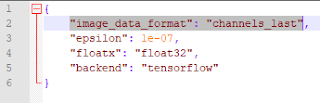# What is the image_data_format parameter in Keras, and why is it important

We've talked about the image_dim_ordering parameter in Keras and why is it important. But since from Keras v2 changed the name of the parameter, I thought of bringing this up again.

As you know, Keras  is a higher-level neural networks library for Python, which is capable of running on top of TensorFlow, CNTK (Microsoft Cognitive Toolkit), or Theano, (and with limited support for MXNet and Deeplearning4j), which Keras refers to as 'Backends'.The 'image_data_format' parameter in the keras.json file
Which backend Keras should use is defined in the keras.json file, which is located at ~/.keras/keras.json in Linux and Mac OS, and at %USERPROFILE%\.keras\keras.json on Windows.

The default keras.json file (default set to TensorFlow) would look like this,
`````` {
"epsilon": 1e-07,
"floatx": "float32",
"image_data_format": "channels_last",
"backend": "tensorflow"
}
``````
The "backend" parameter should either be "tensorflow", "cntk", or "theano". When switching the backend, make sure to switch the "image_data_format" parameter too. For "tensorflow "or "cntk" backends, it should be “channels_last”. For “theano”, it should be “channels_first”.

So, the keras.json for CNTK should look like,
`````` {
"epsilon": 1e-07,
"floatx": "float32",
"image_data_format": "channels_last",
"backend": "cntk"
}
``````

Likewise, the keras,json for Theano would look like this,
`````` {
"epsilon": 1e-07,
"floatx": "float32",
"image_data_format": "channels_first",
"backend": "theano"
}
``````

Why is this image_data_format parameter so important?

The image_data_format parameter affects how each of the backends treat the data dimensions when working with multi-dimensional convolution layers (such as Conv2D, Conv3D, Conv2DTranspose, Copping2D, … and any other 2D or 3D layer). Specifically, it defines where the 'channels' dimension is in the input data.

Both TensorFlow and Theano expects a four dimensional tensor as input. But where TensorFlow expects the 'channels' dimension as the last dimension (index 3, where the first is index 0) of the tensor – i.e. tensor with shape (samples, rows, cols, channels) – Theano will expect 'channels' at the second dimension (index 1) – i.e. tensor with shape (samples, channels, rows, cols). The outputs of the convolutional layers will also follow this pattern.

So, the image_data_format parameter, once set in keras.json, will tell Keras which dimension ordering to use in its convolutional layers.

Mixing up the channels order would result in your models being trained in unexpected ways.

Other than by setting the parameter in keras.json you can manipulate it in the code as well. You can get and set the image_data_format through the keras.backend package.

To get the image_data_format, you can use the image_data_format() function,
`````` from keras import backend as K
print(K.image_data_format())
``````

To set the image_data_format, pass the string either ‘channels_first’ or ‘channels_last’ to set_image_data_format() function.
`````` from keras import backend as K
K.set_image_data_format('channels_first')
``````

You can also set it per layer, using the data_format parameter in the 2D and 3D convolutional layers.
`````` model.add(Conv2D(20, (5, 5), padding="same", input_shape=(height, width, depth), data_format="channels_first"))
``````

When manipulating it programmatically, just make sure to keep track of what you change it in to. Otherwise you might mess up training of your model.

Build Deeper: The Path to Deep Learning

Learn the bleeding edge of AI in the most practical way: By getting hands-on with Python, TensorFlow, Keras, and OpenCV. Go a little deeper...

1.2.